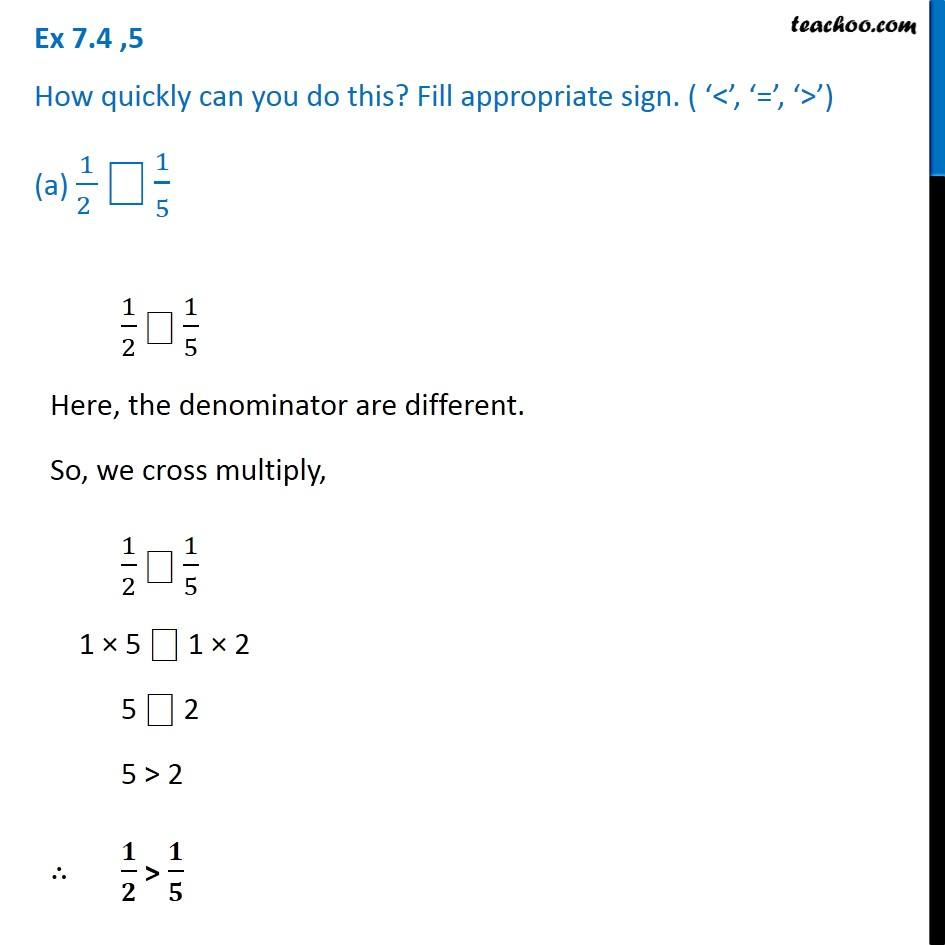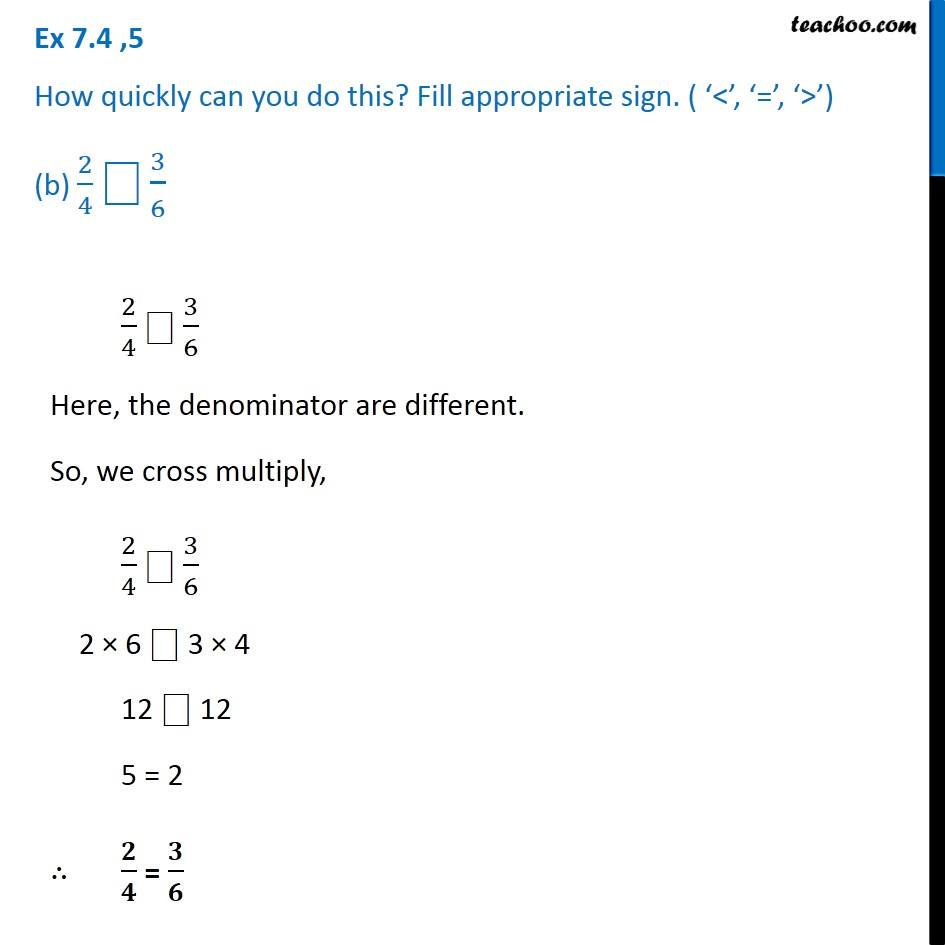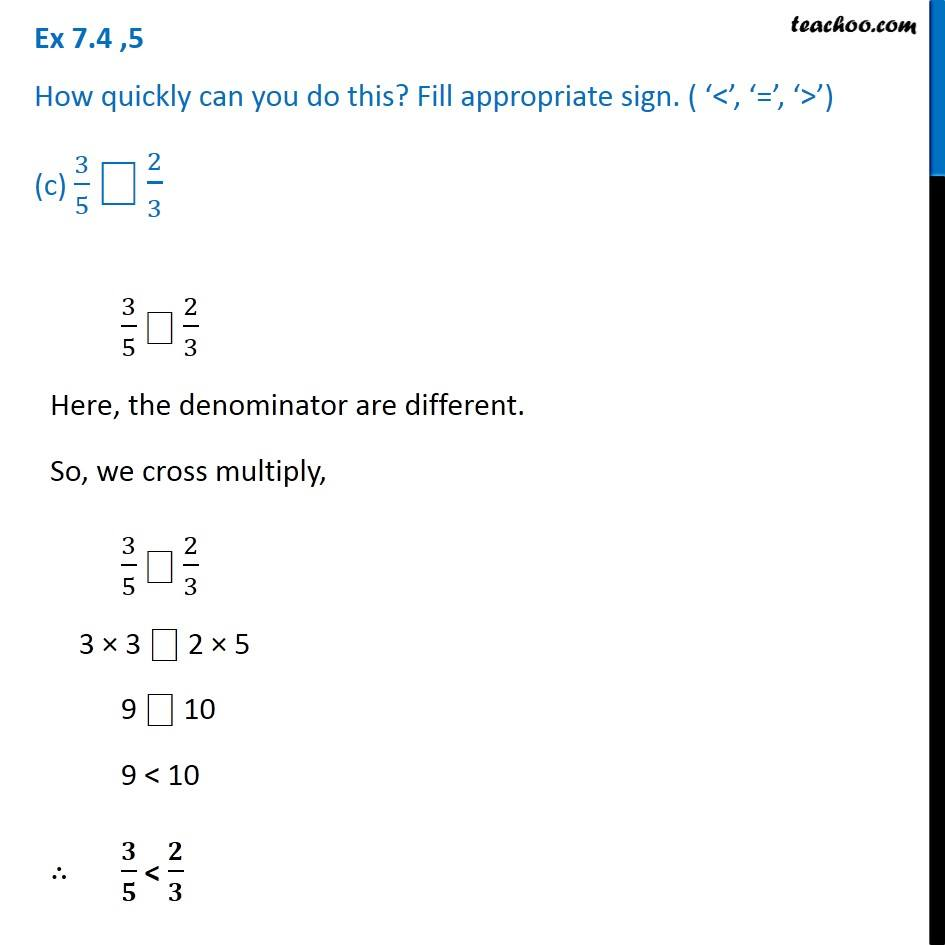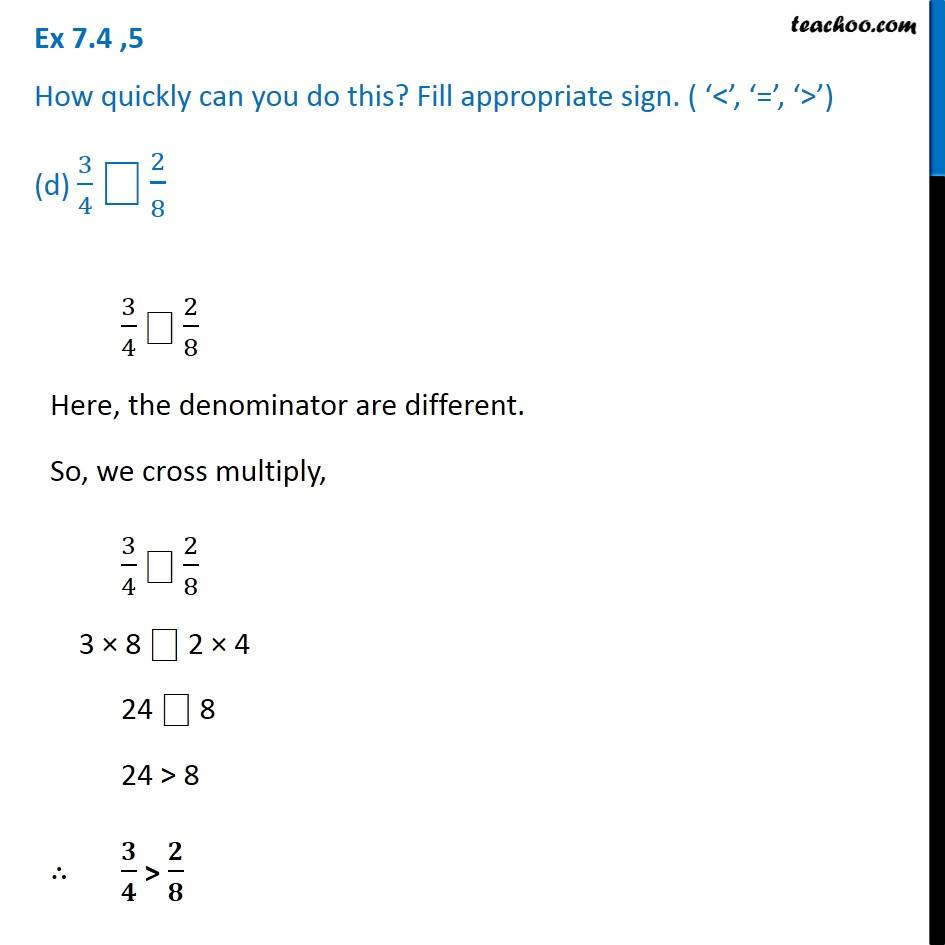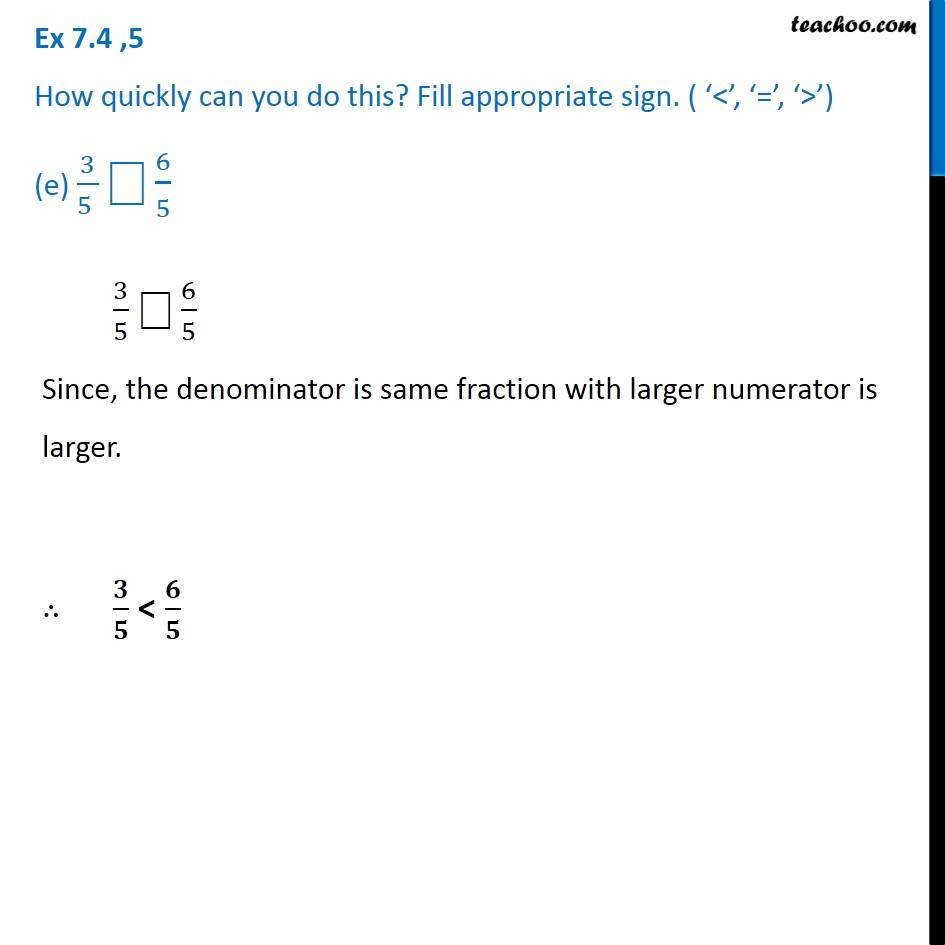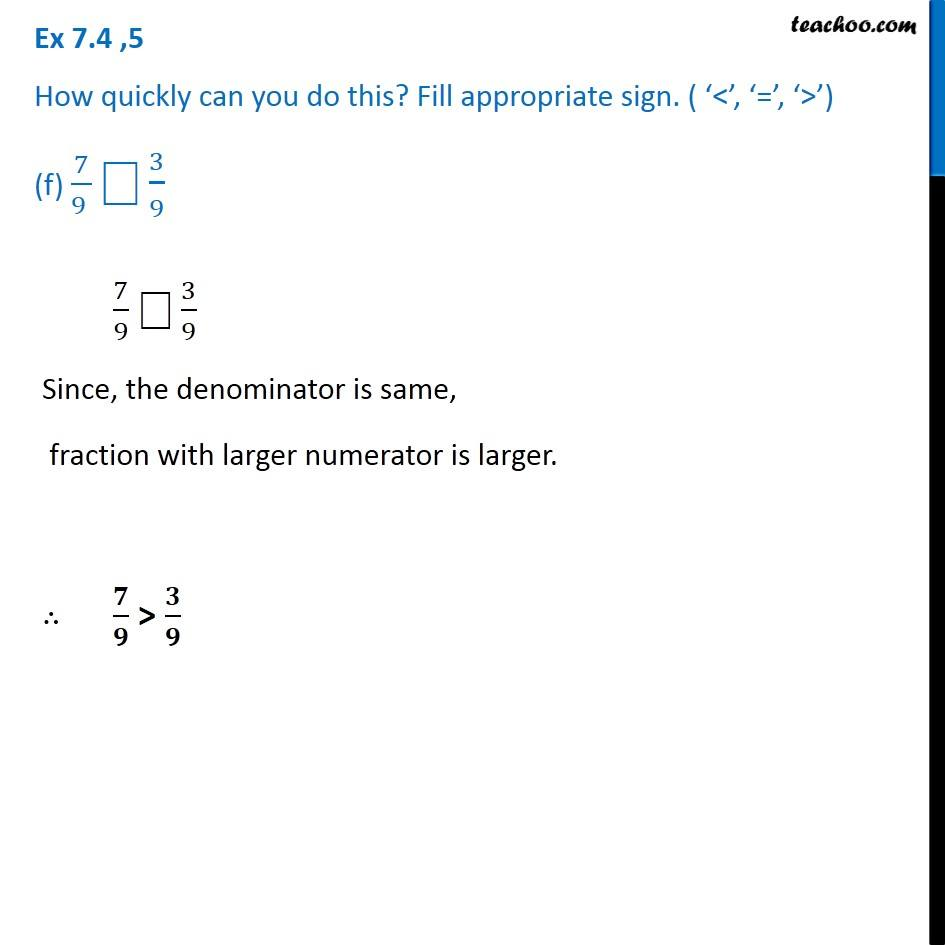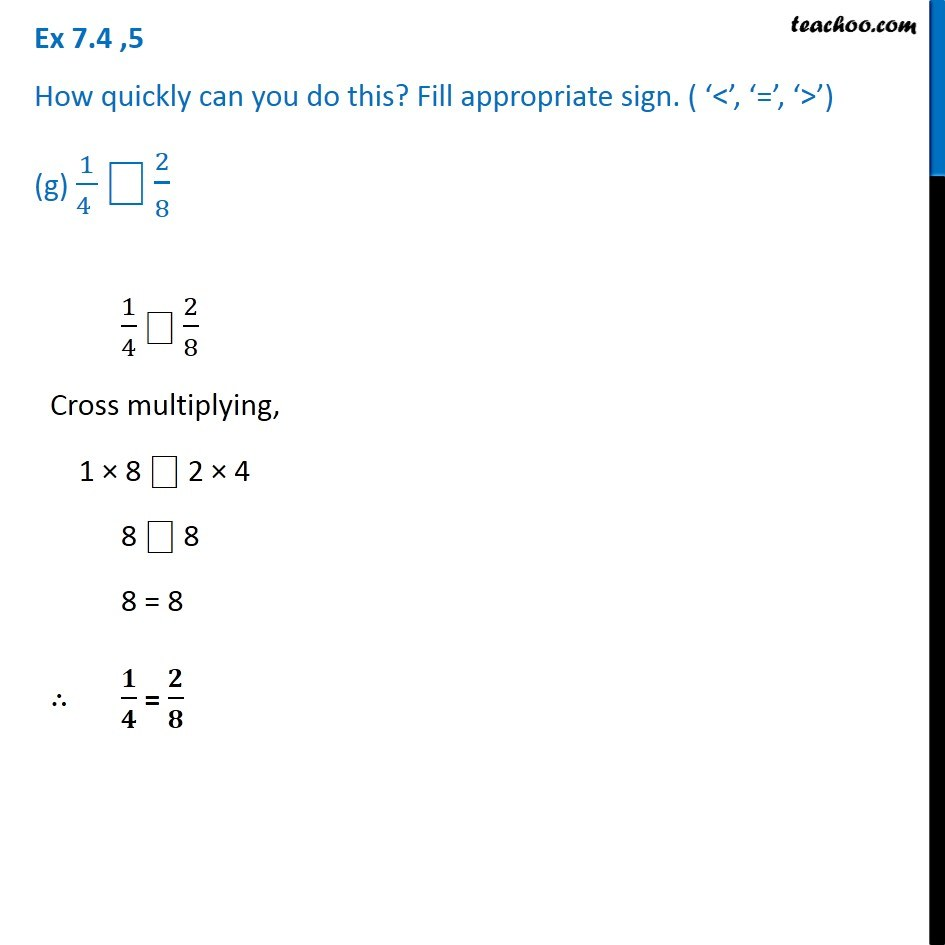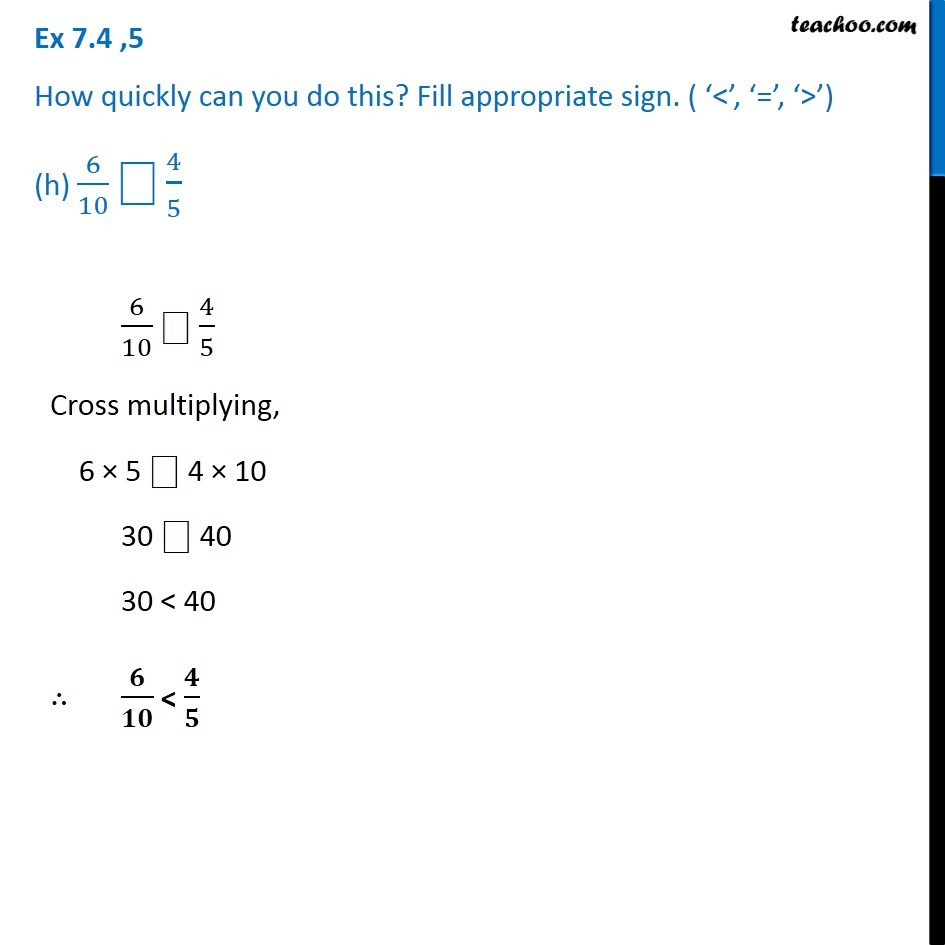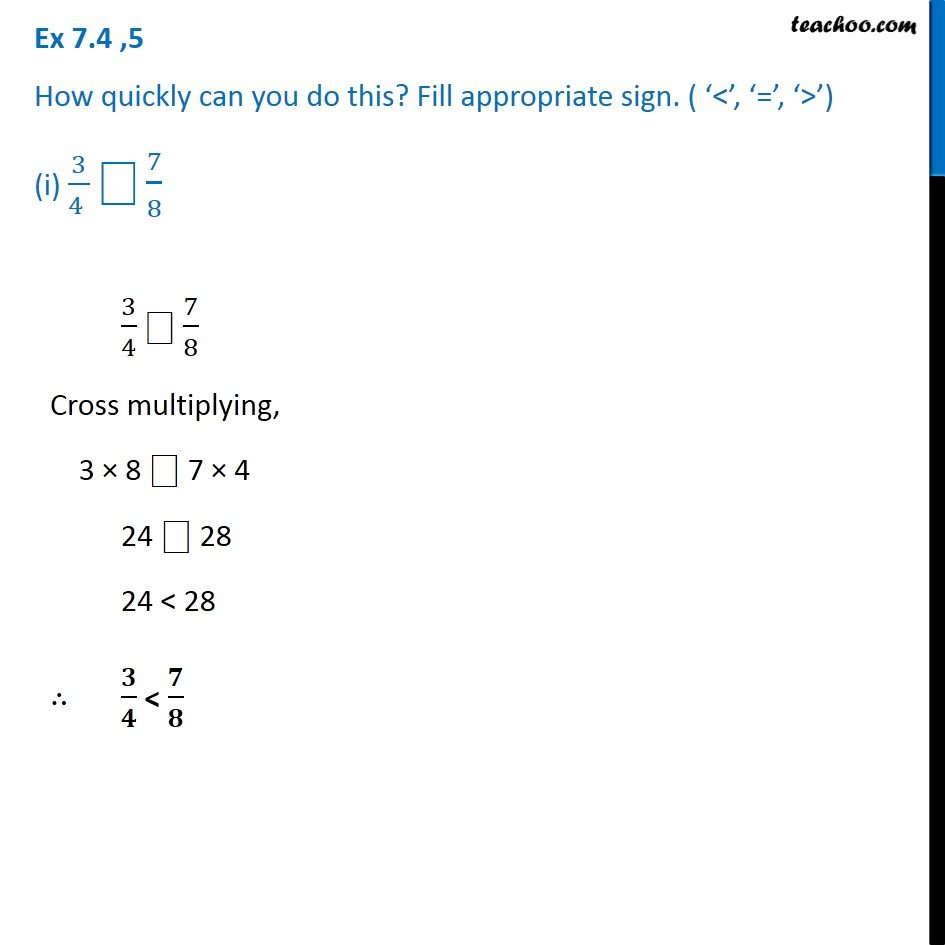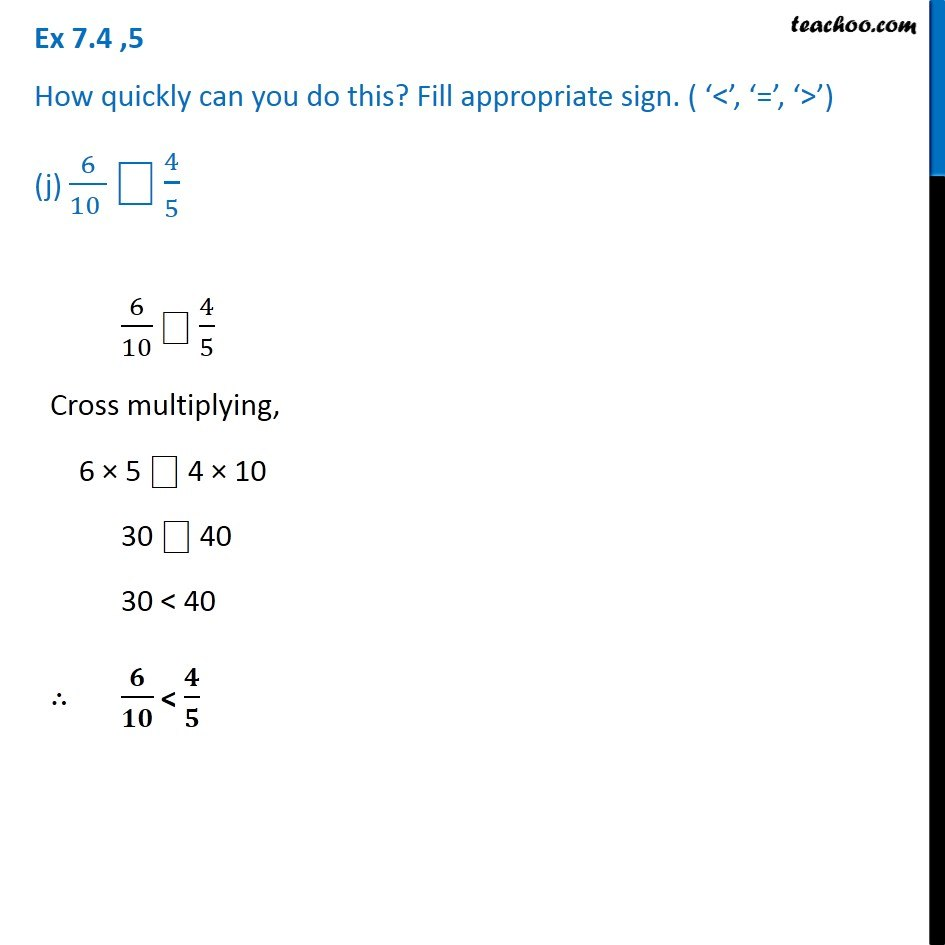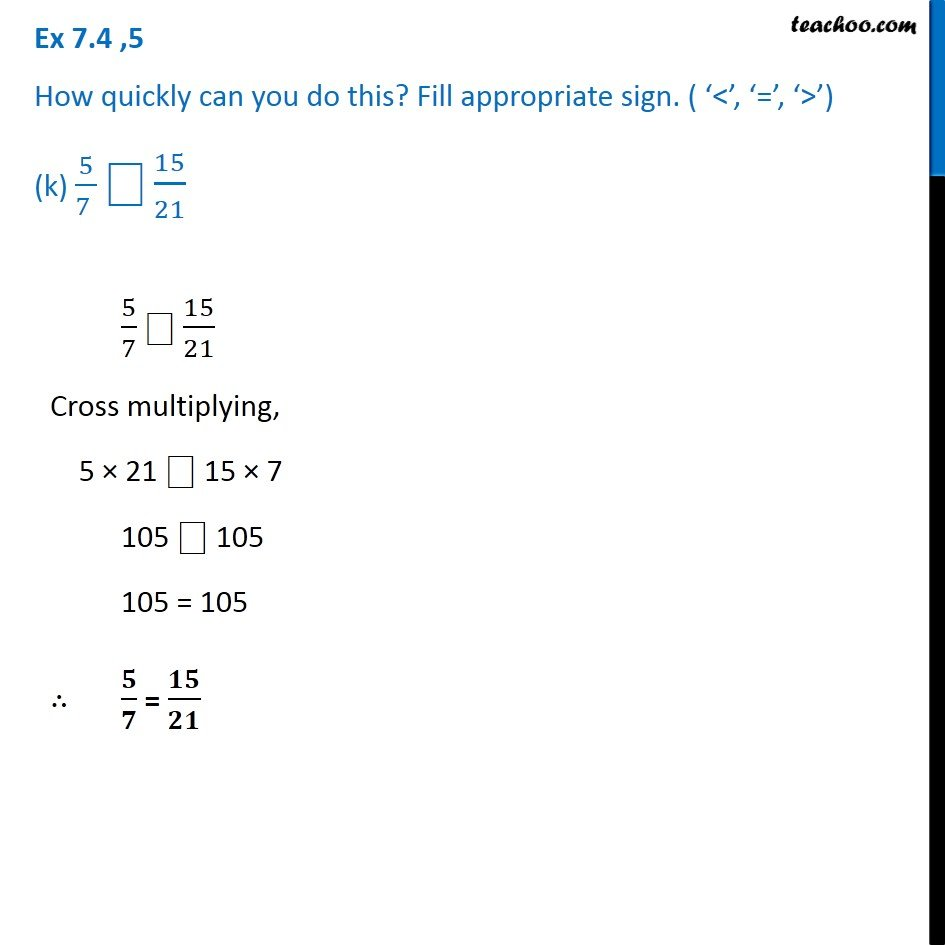1. Chapter 7 Class 6 Fractions
2. Serial order wise
3. Ex 7.4

Transcript

Ex 7.4 ,5 How quickly can you do this? Fill appropriate sign. ( ‘<’, ‘=’, ‘>’) (a) 1/(2 ) ⎕1/5 1/2 ⎕ 1/5 Here, the denominator are different. So, we cross multiply, 1/2 ⎕ 1/5 1 × 5 ⎕ 1 × 2 5 ⎕ 2 5 > 2 ∴ 𝟏/𝟐 > 𝟏/𝟓 Ex 7.4 ,5 How quickly can you do this? Fill appropriate sign. ( ‘<’, ‘=’, ‘>’) (b) 2/4 ⎕3/6 2/4 ⎕ 3/6 Here, the denominator are different. So, we cross multiply, 2/4 ⎕ 3/6 2 × 6 ⎕ 3 × 4 12 ⎕ 12 5 = 2 ∴ 𝟐/𝟒 = 𝟑/𝟔 Ex 7.4 ,5 How quickly can you do this? Fill appropriate sign. ( ‘<’, ‘=’, ‘>’) (c) 3/5 ⎕2/3 3/5 ⎕ 2/3 Here, the denominator are different. So, we cross multiply, 3/5 ⎕ 2/3 3 × 3 ⎕ 2 × 5 9 ⎕ 10 9 < 10 ∴ 𝟑/𝟓 < 𝟐/𝟑 Ex 7.4 ,5 How quickly can you do this? Fill appropriate sign. ( ‘<’, ‘=’, ‘>’) (d) 3/4 ⎕2/8 3/4 ⎕ 2/8 Here, the denominator are different. So, we cross multiply, 3/4 ⎕ 2/8 3 × 8 ⎕ 2 × 4 24 ⎕ 8 24 > 8 ∴ 𝟑/𝟒 > 𝟐/𝟖 Ex 7.4 ,5 How quickly can you do this? Fill appropriate sign. ( ‘<’, ‘=’, ‘>’) (e) 3/(5 ) ⎕6/5 3/5 "⎕" 6/5 Since, the denominator is same fraction with larger numerator is larger. ∴ 𝟑/𝟓 < 𝟔/𝟓 Ex 7.4 ,5 How quickly can you do this? Fill appropriate sign. ( ‘<’, ‘=’, ‘>’) (f) 7/(9 ) ⎕3/9 7/9 "⎕" 3/9 Since, the denominator is same, fraction with larger numerator is larger. ∴ 𝟕/𝟗 > 𝟑/𝟗 Ex 7.4 ,5 How quickly can you do this? Fill appropriate sign. ( ‘<’, ‘=’, ‘>’) (g) 1/(4 ) ⎕2/8 1/4 ⎕ 2/8 Cross multiplying, 1 × 8 ⎕ 2 × 4 8 ⎕ 8 8 = 8 ∴ 𝟏/𝟒 = 𝟐/𝟖 Ex 7.4 ,5 How quickly can you do this? Fill appropriate sign. ( ‘<’, ‘=’, ‘>’) (h) 6/10 ⎕4/5 6/10 ⎕ 4/5 Cross multiplying, 6 × 5 ⎕ 4 × 10 30 ⎕ 40 30 < 40 ∴ 𝟔/𝟏𝟎 < 𝟒/𝟓 Ex 7.4 ,5 How quickly can you do this? Fill appropriate sign. ( ‘<’, ‘=’, ‘>’) (i) 3/(4 ) ⎕7/8 3/4 ⎕ 7/8 Cross multiplying, 3 × 8 ⎕ 7 × 4 24 ⎕ 28 24 < 28 ∴ 𝟑/𝟒 < 𝟕/𝟖 Ex 7.4 ,5 How quickly can you do this? Fill appropriate sign. ( ‘<’, ‘=’, ‘>’) (j) 6/(10 ) ⎕4/5 6/10 ⎕ 4/5 Cross multiplying, 6 × 5 ⎕ 4 × 10 30 ⎕ 40 30 < 40 ∴ 𝟔/𝟏𝟎 < 𝟒/𝟓 Ex 7.4 ,5 How quickly can you do this? Fill appropriate sign. ( ‘<’, ‘=’, ‘>’) (k) 5/(7 ) ⎕15/21 5/7 ⎕ 15/21 Cross multiplying, 5 × 21 ⎕ 15 × 7 105 ⎕ 105 105 = 105 ∴ 𝟓/𝟕 = 𝟏𝟓/𝟐𝟏

Ex 7.4

Chapter 7 Class 6 Fractions
Serial order wise

About the AuthorDavneet Singh
Davneet Singh is a graduate from Indian Institute of Technology, Kanpur. He has been teaching from the past 10 years. He provides courses for Maths and Science at Teachoo.